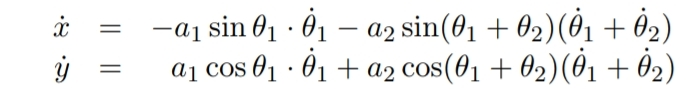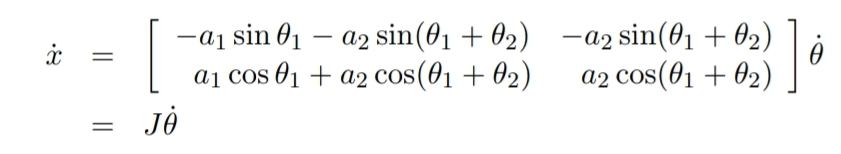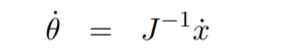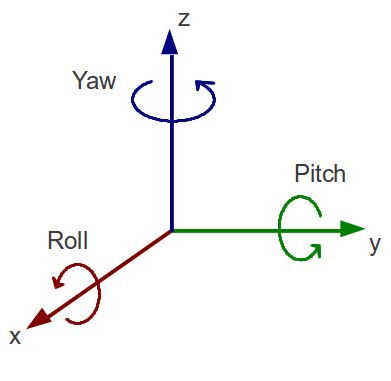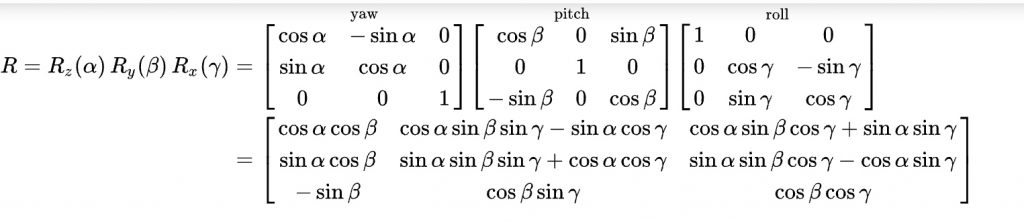# Robot maths

Robotic arm maths: position and velocity

Typically, the manipulator will be able to sense its own position in some manner using internal sensors (position encoders located at joints 1 and 2) that can measure directly the joint angles phi1 and phi2. We also need therefore to express the positions A and B in terms of these joint angles. This leads to the forward kinematics problem which is to determine the position and orientation of the end effector or tool in terms of the joint variables. It is customary to establish a fixed coordinate system, called the world or base frame to which all objects including the manipulator are referenced. In this case we establish the base coordinate frame o0x0y0 at the base of the robot, as shown in Figure 1. The coordinates (x, y) of the tool are expressed in this coordinate frame in which a1 and a2 are the lengths of the two links, respectively. Also the orientation of the tool frame relative to the base frame is given by the direction cosines of the x2 and y2 axes relative to the x0 and y0 axes, that is shown and which we may combine into a rotation matrix (Figure 2).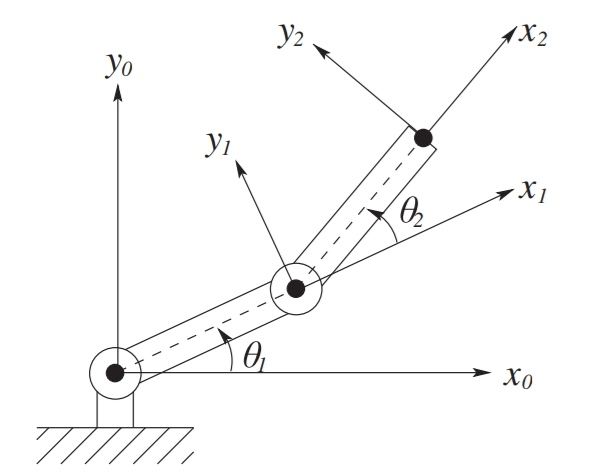Figure 1. Robotic arm with six degrees of freedom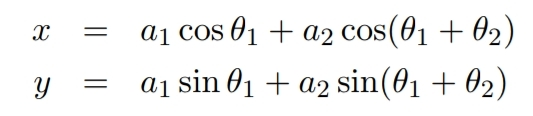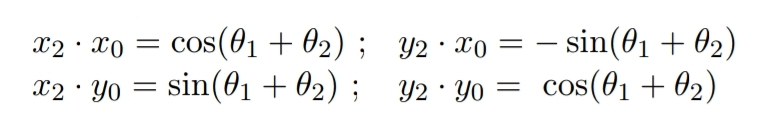Equations in Figure 2 are called the forward kinematic equations for this arm. For a six-DOF robot these equations are quite complex and cannot be written down as easily as for the two-link manipulator. The general procedure establishes coordinate frames at each joint and allows one to transform systematically among theseframes using matrix transformations. The procedure that we use is referred to as the Denavit–Hartenberg convention. We then use homogeneous coordinates and homogeneous transformations to simplify the transformation among coordinate frames.

Concerning velocity kinematics to follow a contour at constant velocity, or at any prescribed velocity, we must know the relationship between the tool velocity and the joint velocities.
In this case we can differentiate Equations in Figure 1 to obtain Figure 2 equations (remember what is a derivative!).